# Tangent lines to circles

In Euclidean plane geometry, a tangent line to a circle is a line that touches the circle at exactly one point, never entering the circle's interior. Roughly speaking, it is a line through a pair of infinitely close points on the circle. Tangent lines to circles form the subject of several theorems, and play an important role in many geometrical constructions and proofs. Since the tangent line to a circle at a point P is perpendicular to the radius to that point, theorems involving tangent lines often involve radial lines and orthogonal circles.

## Tangent lines to one circle

A tangent line t to a circle C intersects the circle at a single point T. For comparison, secant lines intersect a circle at two points, whereas another line may not intersect a circle at all. This property of tangent lines is preserved under many geometrical transformations, such as scalings, rotation, translations, inversions, and map projections. In technical language, these transformations do not change the incidence structure of the tangent line and circle, even though the line and circle may be deformed.

The radius of a circle is perpendicular to the tangent line through its endpoint on the circle's circumference. Conversely, the perpendicular to a radius through the same endpoint is a tangent line. The resulting geometrical figure of circle and tangent line has a reflection symmetry about the axis of the radius.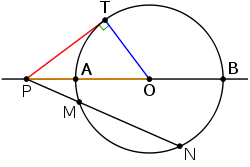By the power-of-a-point theorem, the product of lengths PM·PN for any ray PMN equals to the square of PT, the length of the tangent line segment (red).

No tangent line can be drawn through a point within a circle, since any such line must be a secant line. However, two tangent lines can be drawn to a circle from a point P outside of the circle. The geometrical figure of a circle and both tangent lines likewise has a reflection symmetry about the radial axis joining P to the center point O of the circle. Thus the lengths of the segments from P to the two tangent points are equal. By the secant-tangent theorem, the square of this tangent length equals the power of the point P in the circle C. This power equals the product of distances from P to any two intersection points of the circle with a secant line passing through P.The angle θ between a chord and a tangent is half the arc belonging to the chord.

The tangent line t and the tangent point T have a conjugate relationship to one another, which has been generalized into the idea of pole points and polar lines. The same reciprocal relation exists between a point P outside the circle and the secant line joining its two points of tangency.

If a point P is exterior to a circle with center O, and if the tangent lines from P touch the circle at points T and S, then ∠TPS and ∠TOS are supplementary (sum to 180°).

If a chord TM is drawn from the tangency point T of exterior point P and ∠PTM ≤ 90° then ∠PTM = (1/2)∠TOM.

### Compass and straightedge constructions

It is relatively straightforward to construct a line t tangent to a circle at a point T on the circumference of the circle:

• A line a is drawn from O, the center of the circle, through the radial point T;
• The line t is the perpendicular line to a.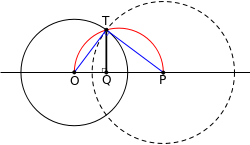Construction of a tangent to a given circle (black) from a given exterior point (P).

Thales' theorem may be used to construct the tangent lines to a point P external to the circle C:

• A circle is drawn centered on the midpoint of the line segment OP, where O is again the center of the circle C.
• The intersection points T1 and T2 are the tangent points for lines passing through P, by the following argument.

The line segments OT1 and OT2 are radii of the circle C; since both are inscribed in a semicircle, they are perpendicular to the line segments PT1 and PT2, respectively. But only a tangent line is perpendicular to the radial line. Hence, the two lines from P and passing through T1 and T2 are tangent to the circle C.

Another method to construct the tangent lines to a point P external to the circle using only a straightedge:

• Draw any three different lines through the given point $P$ that intersect the circle twice.
• Letbe the six intersection points, with the same letter corresponding to the same line and the index 1 corresponding to the point closer to P.
• Let D be the point where the linesandintersect,
• Similarly E for the linesand.
• Draw a line through D and E.
• This line meets the circle at two points, F and G.
• The tangents are the lines PF and PG. 

### Tangent quadrilateral theorem and inscribed circles

A tangential quadrilateral ABCD is a closed figure of four straight sides that are tangent to a given circle C. Equivalently, the circle C is inscribed in the quadrilateral ABCD. By the Pitot theorem, the sums of opposite sides of any such quadrilateral are equal, i.e.,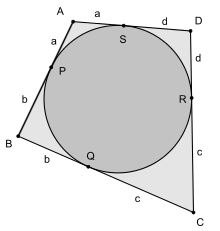This conclusion follows from the equality of the tangent segments from the four vertices of the quadrilateral. Let the tangent points be denoted as P (on segment AB), Q (on segment BC), R (on segment CD) and S (on segment DA). The symmetric tangent segments about each point of ABCD are equal, e.g., BP=BQ=b, CQ=CR=c, DR=DS=d, and AS=AP=a. But each side of the quadrilateral is composed of two such tangent segmentsproving the theorem.

The converse is also true: a circle can be inscribed into every quadrilateral in which the lengths of opposite sides sum to the same value.

This theorem and its converse have various uses. For example, they show immediately that no rectangle can have an inscribed circle unless it is a square, and that every rhombus has an inscribed circle, whereas a general parallelogram does not.

## Tangent lines to two circles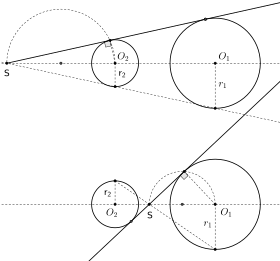The external (above) and internal (below) homothetic center S of the two circles.

For two circles, there are generally four distinct lines that are tangent to both (bitangent) – if the two circles are outside each other – but in degenerate cases there may be any number between zero and four bitangent lines; these are addressed below. For two of these, the external tangent lines, the circles fall on the same side of the line; for the two others, the internal tangent lines, the circles fall on opposite sides of the line. The external tangent lines intersect in the external homothetic center, whereas the internal tangent lines intersect at the internal homothetic center. Both the external and internal homothetic centers lie on the line of centers (the line connecting the centers of the two circles), closer to the center of the smaller circle: the internal center is in the segment between the two circles, while the external center is not between the points, but rather outside, on the side of the center of the smaller circle. If the two circles have equal radius, there are still four bitangents, but the external tangent lines are parallel and there is no external center in the affine plane; in the projective plane, the external homothetic center lies at the point at infinity corresponding to the slope of these lines.

### Outer tangent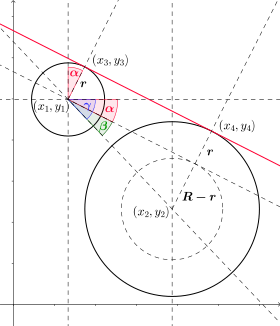Finding outer tangent. Two circles' outer tangents.

The red line joining the pointsandis the outer tangent between the two circles. Given points,the points,can easily be calculated with help of the angle:Here R and r notate the radii of the two circles and the anglecan be computed using basic trigonometry. You havewithand.

### Inner tangent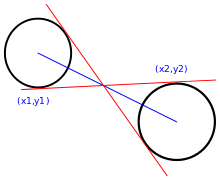Inner tangent. The external tangent lines pass through the internal homothetic center.

An inner tangent is a tangent that intersects the segment joining two circles' centers. Note that the inner tangent will not be defined for cases when the two circles overlap.

### Construction

The bitangent lines can be constructed either by constructing the homothetic centers, as described at that article, and then constructing the tangent lines through the homothetic center that is tangent to one circle, by one of the methods described above. The resulting line will then be tangent to the other circle as well. Alternatively, the tangent lines and tangent points can be constructed more directly, as detailed below. Note that in degenerate cases these constructions break down; to simplify exposition this is not discussed in this section, but a form of the construction can work in limit cases (e.g., two circles tangent at one point).

#### Synthetic geometry

Let O1 and O2 be the centers of the two circles, C1 and C2 and let r1 and r2 be their radii, with r1 > r2; in other words, circle C1 is defined as the larger of the two circles. Two different methods may be used to construct the external and internal tangent lines.

External tangentsConstruction of the outer tangent

A new circle C3 of radius r1  r2 is drawn centered on O1. Using the method above, two lines are drawn from O2 that are tangent to this new circle. These lines are parallel to the desired tangent lines, because the situation corresponds to shrinking both circles C1 and C2 by a constant amount, r2, which shrinks C2 to a point. Two radial lines may be drawn from the center O1 through the tangent points on C3; these intersect C1 at the desired tangent points. The desired external tangent lines are the lines perpendicular to these radial lines at those tangent points, which may be constructed as described above.

Internal tangentsConstruction of the immer tangent

A new circle C3 of radius r1 + r2 is drawn centered on O1. Using the method above, two lines are drawn from O2 that are tangent to this new circle. These lines are parallel to the desired tangent lines, because the situation corresponds to shrinking C2 to a point while expanding C1 by a constant amount, r2. Two radial lines may be drawn from the center O1 through the tangent points on C3; these intersect C1 at the desired tangent points. The desired internal tangent lines are the lines perpendicular to these radial lines at those tangent points, which may be constructed as described above.

#### Analytic geometry

Let the circles have centres c1 = (x1,y1) and c2 = (x2,y2) with radius r1 and r2 respectively. Expressing a line by the equationwith the normalization a2 + b2 = 1, then a bitangent line satisfies:

ax1 + by1 + c = r1 and
ax2 + by2 + c = r2.

Solving forby subtracting the first from the second yields

aΔx + bΔy = Δr

where Δx = x2  x1, Δy = y2  y1 and Δr = r2  r1.

Ifis the distance from c1 to c2 we can normalize by X = Δx/d, Y = Δy/d and R = Δr/d to simplify equations, yielding the equations aX + bY = R and a2 + b2 = 1, solve these to get two solutions (k = ±1) for the two external tangent lines:

a = RX  kY√(1  R2)
b = RY + kX√(1  R2)
c = r1  (ax1 + by1)

Geometrically this corresponds to computing the angle formed by the tangent lines and the line of centers, and then using that to rotate the equation for the line of centers to yield an equation for the tangent line. The angle is computed by computing the trigonometric functions of a right triangle whose vertices are the (external) homothetic center, a center of a circle, and a tangent point; the hypotenuse lies on the tangent line, the radius is opposite the angle, and the adjacent side lies on the line of centers.

(X, Y) is the unit vector pointing from c1 to c2, while R iswhereis the angle between the line of centers and a tangent line.is then(depending on the sign of, equivalently the direction of rotation), and the above equations are rotation of (X, Y) byusing the rotation matrix:k = 1 is the tangent line to the right of the circles looking from c1 to c2.
k = 1 is the tangent line to the right of the circles looking from c2 to c1.

The above assumes each circle has positive radius. If r1 is positive and r2 negative then c1 will lie to the left of each line and c2 to the right, and the two tangent lines will cross. In this way all four solutions are obtained. Switching signs of both radii switches k = 1 and k = 1.

#### Vectors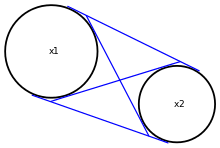Finding outer tangent. Circle tangents.

In general the points of tangency t1 and t2 for the four lines tangent to two circles with centers v1 and v2 and radii r1 and r2 are given by solving the simultaneous equations:These equations express that the tangent line, which is parallel tois perpendicular to the radii, and that the tangent points lie on their respective circles.

These are four quadratic equations in two two-dimensional vector variables, and in general position will have four pairs of solutions.

### Degenerate cases

Two distinct circles may have between zero and four bitangent lines, depending on configuration; these can be classified in terms of the distance between the centers and the radii. If counted with multiplicity (counting a common tangent twice) there are zero, two, or four bitangent lines. Bitangent lines can also be generalized to circles with negative or zero radius. The degenerate cases and the multiplicities can also be understood in terms of limits of other configurations – e.g., a limit of two circles that almost touch, and moving one so that they touch, or a circle with small radius shrinking to a circle of zero radius.

• If the circles are outside each other (), which is general position, there are four bitangents.
• If they touch externally at one point () – have one point of external tangency – then they have two external bitangents and one internal bitangent, namely the common tangent line. This common tangent line has multiplicity two, as it separates the circles (one on the left, one on the right) for either orientation (direction).
• If the circles intersect in two points (), then they have no internal bitangents and two external bitangents (they cannot be separated, because they intersect, hence no internal bitangents).
• If the circles touch internally at one point () – have one point of internal tangency – then they have no internal bitangents and one external bitangent, namely the common tangent line, which has multiplicity two, as above.
• If one circle is completely inside the other () then they have no bitangents, as a tangent line to the outer circle does not intersect the inner circle, or conversely a tangent line to the inner circle is a secant line to the outer circle.

Finally, if the two circles are identical, any tangent to the circle is a common tangent and hence (external) bitangent, so there is a circle's worth of bitangents.

Further, the notion of bitangent lines can be extended to circles with negative radius (the same locus of points,but considered "inside out"), in which case if the radii have opposite sign (one circle has negative radius and the other has positive radius) the external and internal homothetic centers and external and internal bitangents are switched, while if the radii have the same sign (both positive radii or both negative radii) "external" and "internal" have the same usual sense (switching one sign switches them, so switching both switches them back).

Bitangent lines can also be defined when one or both of the circles has radius zero. In this case the circle with radius zero is a double point, and thus any line passing through it intersects the point with multiplicity two, hence is "tangent". If one circle has radius zero, a bitangent line is simply a line tangent to the circle and passing through the point, and is counted with multiplicity two. If both circles have radius zero, then the bitangent line is the line they define, and is counted with multiplicity four.

Note that in these degenerate cases the external and internal homothetic center do generally still exist (the external center is at infinity if the radii are equal), except if the circles coincide, in which case the external center is not defined, or if both circles have radius zero, in which case the internal center is not defined.

### Applications

#### Belt problem

Main article: Belt problem

The internal and external tangent lines are useful in solving the belt problem, which is to calculate the length of a belt or rope needed to fit snugly over two pulleys. If the belt is considered to be a mathematical line of negligible thickness, and if both pulleys are assumed to lie in exactly the same plane, the problem devolves to summing the lengths of the relevant tangent line segments with the lengths of circular arcs subtended by the belt. If the belt is wrapped about the wheels so as to cross, the interior tangent line segments are relevant. Conversely, if the belt is wrapped exteriorly around the pulleys, the exterior tangent line segments are relevant; this case is sometimes called the pulley problem.

## Tangent lines to three circles: Monge's theorem

Main article: Monge's theorem

For three circles denoted by C1, C2, and C3, there are three pairs of circles (C1C2, C2C3, and C1C3). Since each pair of circles has two homothetic centers, there are six homothetic centers altogether. Gaspard Monge showed in the early 19th century that these six points lie on four lines, each line having three collinear points.

## Problem of Apollonius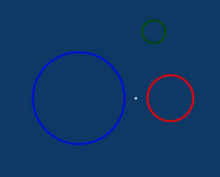Animation showing the inversive transformation of an Apollonius problem. The blue and red circles swell to tangency, and are inverted in the grey circle, producing two straight lines. The yellow solutions are found by sliding a circle between them until it touches the transformed green circle from within or without.

Many special cases of Apollonius's problem involve finding a circle that is tangent to one or more lines. The simplest of these is to construct circles that are tangent to three given lines (the LLL problem). To solve this problem, the center of any such circle must lie on an angle bisector of any pair of the lines; there are two angle-bisecting lines for every intersection of two lines. The intersections of these angle bisectors give the centers of solution circles. There are four such circles in general, the inscribed circle of the triangle formed by the intersection of the three lines, and the three exscribed circles.

A general Apollonius problem can be transformed into the simpler problem of circle tangent to one circle and two parallel lines (itself a special case of the LLC special case). To accomplish this, it suffices to scale two of the three given circles until they just touch, i.e., are tangent. An inversion in their tangent point with respect to a circle of appropriate radius transforms the two touching given circles into two parallel lines, and the third given circle into another circle. Thus, the solutions may be found by sliding a circle of constant radius between two parallel lines until it contacts the transformed third circle. Re-inversion produces the corresponding solutions to the original problem.

## Generalizations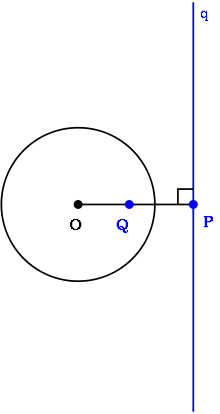The concept of a tangent line and tangent point can be generalized to a pole point Q and its corresponding polar line q. The points P and Q are inverses of each other with respect to the circle.

The concept of a tangent line to one or more circles can be generalized in several ways. First, the conjugate relationship between tangent points and tangent lines can be generalized to pole points and polar lines, in which the pole points may be anywhere, not only on the circumference of the circle. Second, the union of two circles is a special (reducible) case of a quartic plane curve, and the external and internal tangent lines are the bitangents to this quartic curve. A generic quartic curve has 28 bitangents.

A third generalization considers tangent circles, rather than tangent lines; a tangent line can be considered as a tangent circle of infinite radius. In particular, the external tangent lines to two circles are limiting cases of a family of circles which are internally or externally tangent to both circles, while the internal tangent lines are limiting cases of a family of circles which are internally tangent to one and externally tangent to the other of the two circles.

In Möbius or inversive geometry, lines are viewed as circles through a point "at infinity" and for any line and any circle, there is a Möbius transformation which maps one to the other. In Möbius geometry, tangency between a line and a circle becomes a special case of tangency between two circles. This equivalence is extended further in Lie sphere geometry.

## References

1. Alexander Bogomolny, "When A Quadrilateral Is Inscriptible?" at Cut-the-knot
2. Paul Kunkel. "Tangent circles". Whistleralley.com. Retrieved 2008-09-29.
3. Libeskind, Shlomo (2007), Euclidean and Transformational Geometry: A Deductive Inquiry, pp. 110112 (online copy, p. 110, at Google Books)
4. Kunkel, Paul (2007), "The tangency problem of Apollonius: three looks" (PDF), BSHM Bulletin: Journal of the British Society for the History of Mathematics, 22 (1): 34–46, doi:10.1080/17498430601148911